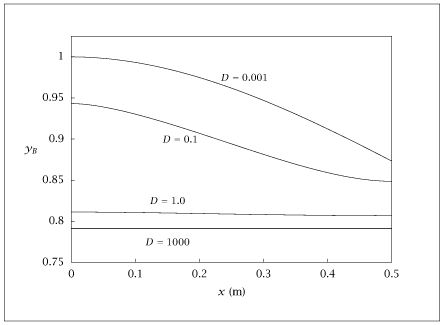## Figure 8.37:

### Yield of desired product B versus reactor length for different dispersion numbers.## Code for Figure 8.37

### main.m

```
1
2
3
4
5
6
7
8
9
10
11
12
13
14
15
16
17
18
19
20
21
22
23
24
25
26
27
28
29
30
31
32
33
34
35
36
37
38
39
40
41
42
43
44
45
46
47
48
49
50
51
52
53
54
55
56
57
58
59
60
61
62
63
64
65
66
67
68
69
70
71
72
73
74
75
76
77
78
79
80
81% Copyright (C) 2001, James B. Rawlings and John G. Ekerdt
%
% This program is free software; you can redistribute it and/or
% modify it under the terms of the GNU General Public License as
% published by the Free Software Foundation; either version 2, or (at
% your option) any later version.
%
% This program is distributed in the hope that it will be useful, but
% WITHOUT ANY WARRANTY; without even the implied warranty of
% MERCHANTABILITY or FITNESS FOR A PARTICULAR PURPOSE.  See the GNU
% General Public License for more details.
%
% You should have received a copy of the GNU General Public License
% along with this program; see the file COPYING.  If not, write to
% the Free Software Foundation, 59 Temple Place - Suite 330, Boston,
% MA 02111-1307, USA.
%
% Revised 8/16/2018

p.k1 = 1;
p.k2 = 1;
p.v =  1;
%p.D = 1000;
%p.D = 1;
p.caf = 1;
p.cbf = 0;
length = 0.5;

ncolpt = 50;
[col.z, col.A, col.B, col.Q] = colloc(ncolpt - 2,'left','right');
col.z = col.z*length;
col.A = col.A/length;
col.B = col.B/(length*length);

Dvec = [1000; 1; 0.1; 1e-3];
nD = size(Dvec, 1);
table(1:nD) = {[]};

for i = 1:nD
p.D = Dvec(i);
x0 = [p.caf*ones(ncolpt,1); p.caf*ones(ncolpt,1)];
opts = optimset('TolFun', 1e-16, 'TolX', 1e-10);
[x, fval, info] = fsolve(@(x) pfrcol(x, ncolpt, p, col), x0, opts);
fsolve_failed = info <= 0;

if  (fsolve_failed)
fprintf ('warning, fsolve failed, info = %d\n', info);
i, p.D, x0
fprintf('i= %g \n',i);
end

ca = x(1:ncolpt);
cb = x(ncolpt+1:2*ncolpt);
yield = cb ./ (p.caf - ca);
conv  = (p.caf - ca) ./ (p.caf);
table(i) = {[col.z conv yield]};
end

save dispersedpfr.dat table;

if (~ strcmp (getenv ('OMIT_PLOTS'), 'true')) % PLOTTING
subplot (2, 1, 1);
hold on

for i = 1:nD
plot (table{i}(:,1),table{i}(:,2))
end

% TITLE dispersedpfr_1

subplot (2, 1, 2);
hold on

for i = 1:nD
plot (table{i}(:,1),table{i}(:,3))
end

hold off
% TITLE dispersedpfr_2
end % PLOTTING

```

### pfrcol.m

```
1
2
3
4
5
6
7
8
9
10
11
12
13
14
15function res = pfrcol(x, ncolpt, p, col)
% express the diff eq at every collocation point
ca = x(1:ncolpt);
cb = x(ncolpt+1:2*ncolpt);
Rab  = [-p.k1*ca; p.k1*ca - 2*p.k2*cb.^2];
first  = [col.A*ca; col.A*cb];
second = [col.B*ca; col.B*cb];
res = -p.v*first + p.D*second + Rab;

% write over the first and last points with the
% boundary conditions
res(1) = p.v*ca(1) - p.D*first(1) - p.v*p.caf;
res(ncolpt) = first(ncolpt);
res(ncolpt+1)= p.v*cb(1) - p.D*first(ncolpt + 1) - p.v*p.cbf;
res(2*ncolpt) = first(2*ncolpt);

```